# Anova Statistics

Question:

The Texas Transportation Institute at Texas A and M University conducted a survey to determine the number of hours per year drivers waste sitting in traffic of 75 urban areas studied; the most jammed urban area was Los Angeles where drivers wasted an average of 90 hours per year. Other jammed urban areas included Denver, Miami and San Francisco. Assume sample data for six drivers in each of these cities show the following number of hour’s waster per year sitting in traffic.

#### Sample data for six drivers:

DenverMiamiSan Francisco
706665
627062
715574
586569
575663
666675
a. Compute the sample mean hours wasted per year for each of these urban areas.
b. Using a + .05, test for significant differences among the population mean wasted time for these three urban areas. What is the p-value? What is your conclusion?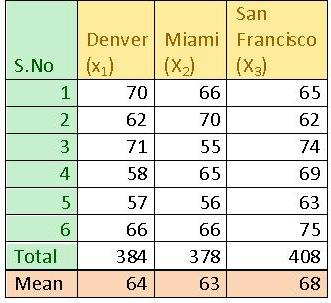Mean of Denver =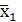=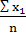=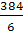= 64
Similarly the other for the other cities can be calculated. Hence we have
Ø Mean of Denver = 64 hours
Ø Mean of Miami = 63 hours
Ø Mean of San Francisco = 68 hoursIn order to test whether there is difference in the mean hours wasted by the car drivers, we have to perform as ANOVA (Analysis of Variance).

Null hypothesis:
H0: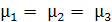That is there is no significance difference among the mean hours wasted by the drivers in the three cities.

Alternate hypothesis:

H1: Not all population means are equal.
That is there is significance difference among the mean hours wasted by the drivers in the three cities.
The Analysis of Variance is carried out using Microsoft Excel. The steps to be followed in Microsoft Excel are as follows:

• Select ‘Data’ from the main menu. Then click ‘Data Analysis’.
• Select ‘ANOVA – one factor’ from the analysis tools.
• Input the given data range in ‘input range’.
• Then click ‘OK’ to get the result.

The above mentioned steps are followed using Microsoft Excel and the output is given below:

 Anova: Single Factor SUMMARY Groups Count Sum Average Variance Denvver 6 384 64 35.6 Miami 6 378 63 36.8 San Francisco 6 408 68 31.2
 ANOVA Source of Variation SS df MS F P-value F crit Between Groups 84 2 42 1.216216 0.323966 3.68232 Within Groups 518 15 34.53333333 Total 602 17

Hence the P-value corresponding to F test statistic is 0.323966.

Statistical decision:
From the Anova table, we see that that P-value (0.323966) corresponding to the F test statistic is greater than 0.05 (level of significance). Hence we do not reject the null hypothesis at 0.05 level of significance.

i.e:, there is no significance difference among the mean hours wasted by the drivers in the three cities.